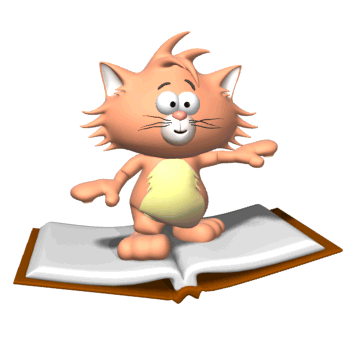• Classroom ProceduresSupplies Needed for 6th Grade Math:
1.  A notebook designated just for math
2. A pencil
3. Highlighter (optional)
 1.  Students will have a "Problem of the Day" to complete when they walk into the room.  The problem will be written on the board.  Students are to copy the problem into their notebook and solve it.    2.  All chapters in the math textbook are broken down into 6-8 lessons.  There is only one book, split into two volumes.  This book can be written in.  There are no more hard cover textbooks for math.   *Note:  Not all chapters/lessons are followed in order.  3.  Usually 1-2 class days are spent on each lesson, depending on each lesson's difficulty.  More time will be used if necessary.  Students are given notes for every lesson.  These notes include vocabulary, procedures, and example problems.    *Taking notes is very important so they always have example problems to look at.    4.  An assignment is given 3-4 days a week to go along with the lesson taught.  This assignment usually becomes homework if it is not finished during the class period (no homework on Fridays). Assignments come from the textbook or activity sheets.  All assignments should be written into their agenda and the agenda should be signed by the parent at least once a week.   *Most homework assignments will be an individual grade.  Students will receive a 100% if the assignment is fully completed.  If the assignment is incomplete or not done at all, the student will receive a 0%.  5.  Students will also use a Coach book from time to time.  This book may not leave the classroom.  It is used to reinforce concepts taught from the textbook and to show students the different types of problems they may see on the PSSA test.  Class notes will be even more important since students cannot take the book home to study.